## Simple Interest Calculator: Bonds, Notes

### Learning Outcomes

• Calculate simple interest over time
• Analyze and compare interest in real-world applications

Savings doesn’t mean just stuffing your money in a mattress or locking it up in a box. When you place your money into a savings product, you are actually lending your money to the bank or organization that holds it. The interest earned on your savings is the fee you get for letting the bank or organization use your money for a time. Some savings products are riskier than others. Generally, the riskier the venture, the more attractive the interest rate they offer to use your money. Look for savings instruments issued by established banks and organizations who’ve had excellent track records for 10 years or longer for the safest options.

A few types of savings instruments pay simple interest but most will compound interest monthly or daily over the course of a year. You’ll look at the math behind both types of interest in this section by creating your own spreadsheet to calculate and compare the returns on different accounts.

## Simple Interest: Bonds and Notes

Bonds and notes are types of savings instruments issued by companies and governments to fund operations or growth. They are offered in various terms at various rates, and some make riskier investments than others. The most secure type of savings instruments possible are sold by the U.S. government. Treasury notes, and bonds are a type of debt with pre-defined lengths of time, called maturity terms, for which you leave your money in the investment. The interest paid semi-annually or annually is called a coupon. Notes mature in terms between one and ten years. Treasury bonds are issued for 30 year terms.

Treasury bills and other zero-coupon bonds do not pay interest but are instead sold to you at a deep discount, paying the full face value at maturity. The amount of the discount becomes your interest earned. Treasury bills mature in less than one year. All of the U.S. government products are sold online through the U.S. Treasury, a bank, broker, or dealer. Treasury Direct is a U.S. government website where you can learn more about these products and purchase and redeem them electronically.

As we saw earlier in the text, simple interest is a percentage of money borrowed that is paid back annually.

### order matters with calculations

Remember to apply the order of operations to any calculation you do.

Handle numbers inside grouping symbols first, then exponents. Then, multiply or divide as you encounter them from left to right. Finally, do any addition or subtraction at the end.

### Simple Interest over Time

$A={{P}_{0}}\left(1+\left(\frac{r}{k}\right)kt\right)$

• $A$ is the amount of money in the account at the end of the $t^{\text{th}}$ year (the account balance including principal plus interest)
• \begin{align}{{P}_{0}}\\\end{align} is the principal (starting amount)
• $r$ is the annual interest yield in decimal form
• $k$ is the number of periods over which the interest is paid during the year
• $t$ is the number of years the principal remains invested in the account

Note that when the interest is paid once at the end of the year, the formula is

$A={{P}_{0}}\left(1+r\right)$

Say you deposit $1000 into a savings account paying 2.5% annual interest that matures in 5 years. How much money will you have (principal plus interest) at the end of the 5 year term? ### Spreadsheet Hands-On: Create a Simple Interest Calculator ### Step 1: Design the work area Open a new blank worksheet and save it somewhere safe. You should save your work frequently as you go or turn on an auto-save feature if available. 1. Double-click on the word “Sheet1” on the tab at the bottom of the worksheet and rename it “Simple Interest.” Keep all the calculators you make in this module in one workbook. At the end, you’ll have a handy reference to use for your own financial planning. 2. In the upper left hand corner, create the following cells. 1. A1: Simple Interest Calculator 2. A3: Principal 3. A4: Rate 4. A5: Periods 5. A6: Years 6. A7: leave this cell blank 7. A8: Balance 3. Shade A3, A4, A6, and A8 green by selecting the cells then clicking on the paint bucket and choosing a color. ### Step 2: Create the formulas Recall the formula $A={{P}_{0}}\left(1+\left(\frac{r}{k}\right)kt\right)$. In this case, the number of periods is permitted to fluctuate, but will normally be given as 1. 1. Type the formula into cell B8. To type a formula or a function, always begin by typing the equal sign. This signals to the spreadsheet that it should calculate what follows rather than simply storing it. 2. The template for the formula is A=P(1+(r/k)kt). You may find it helpful to type this into a nearby cell as a guide as you build the formula from the cells containing the values you’ll need. 3. You’ll need numbers populated in the cells. Otherwise, you’ll get an error when you type in the formula. Use the the numbers from the above example. That way, you can check to be sure your formula returns the correct balance. 1. You purchase a$1000 bond paying 2.5% annual simple interest that matures in 5 years.
2. Then $A={{P}_{0}}\left(1+\left(\frac{r}{k}\right)kt\right)=1000\left(1+\left(\frac{.025}{1}\right)\left(1\ast 5\right)\right)=1000\left(1+.025\ast 5\right)=1125$
3. Type these numbers into cells B3, B4, B5, and B6: 1000, 0.025, 1, and 5.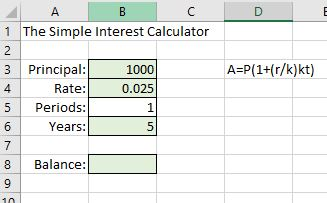4. Into cell B8, type the formula =B3*(1+(B4/B5)*(B5*B6)), then enter. The balance should be populated with the number you calculated by hand: 1125.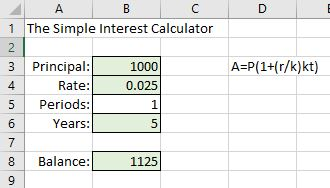5. You can format the Balance cell to represent money if you wish. Right-click on cell B8 and choose the $format. You can also click in the Number tab in the ribbon above the sheet to change the formatting of the cell. 6. Test your formula to see that it works for multiple periods. You know that 2.5% interest on$1000 is $\left(0.025\right)\left(1000\right)=25$. If you were paid this interest twice a year, you’d receive $12.50 per each of six months. Receiving$12.50 twice a year for 5 years should result in the same balance at the end: $1000+12.50\ast 10\text{ periods }=1125.00$. Change the Periods in your spreadsheet to 2 and enter. The balance should remain $1,125.00.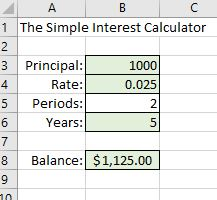Let’s add some more functionality to the spreadsheet. If you had purchased a bond, only the$1000 would be returned at the end of the bond maturity term. You would receive the interest coupons periodically. Let’s set up an area to calculate just the interest earned.

1. In cell A10, type “Interest”.
2. In cell B10, type the formula =B3*B4. That will multiply the Principal times the Rate and will return the simple annual interest on the bond, $25 on our$1000 principal.
3. In cell C10, type “per year”
4. In cell B11, calculate the period interest by typing =B3*(B4/B5). For our example, this will be $\left(1000\right)\left(\dfrac{0.025}{2}\right)=12.50$
5. In cell C11, type =B5 & ” times per year”. This formula uses the & to combine the cell reference for periods with the text “times per year.”
6. In cell B12, total the interest by multiplying the formula in B11 by periods*years: =B3*(B4/B5)*(B5*B6). It should look like the image below when you have finished.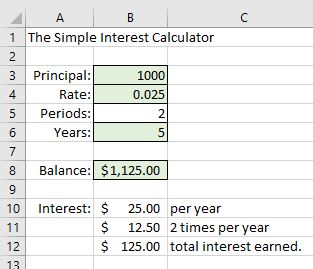7. Play around with the numbers. Try changing the number of periods to see how the amount and times per year change but the interest per year and total interest earned does not change.

Now let’s include the functionality to use our formula to answer the questions like how long should the maturity period be or how large a bond should I purchase to earn a particular amount of interest. Since the answers to these questions won’t depend on the number of periods, we’ll use the formula $A={{P}_{0}}\left(1+rt\right)$. Let’s list that formula in the spreadsheet as a guideline, solved for each of its variables. Then store the formulas to use.

\begin{align}&A={{P}_{0}}\left(1+rt\right)\\&{{P}_{0}=\dfrac{A}{\left(1+rt\right)}}\\&r=\dfrac{A-{{P}_{0}}}{t{{P}_{0}}}\\&t=\dfrac{A-{{P}_{0}}}{r{{P}_{0}}}\end{align}

### How were those formulas solved?

1. Copy cells A3-B8 into the spaces in cells A14 – B19. Click on cell B19 (it should currently show $1,125.00) and delete the formula from it. Empty cells B14 through B17 as well. Finally, move all the cells together and label them as shown below. Include the additional labels underneath. We’ll type the formulas into the spaces next to them. (To subscript the zero in ${{P}_{0}}$, type P0, select the 0, open the Font tab in the ribbon above the spreadsheet, and click subscript.)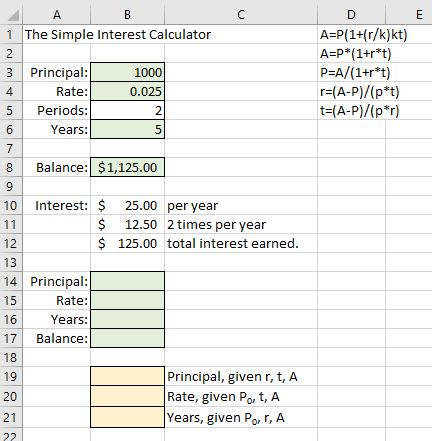2. Into cell B19, type =B17/(1+B15*B16) 3. Into cell B20, type =(B17-B14)/(B14*B16) 4. Into cell B21, type =(B17-B14)/(B14*B15) 5. Now we have the functionality to calculate any one of the variables given all the others. To test that it works, enter the numbers we used in B3 – B6 into the new green shaded cells: 1000, 0.025, 5, and 1125. You may change your formatting as desired between number and$ in the Number tab in the ribbon above the spreadsheet.

Finally, we’ll add the simple interest calculations for one year of investment.

1. Add the labels shown below for cells A23 – C25. The formulas have been listed next to each of their respective cells.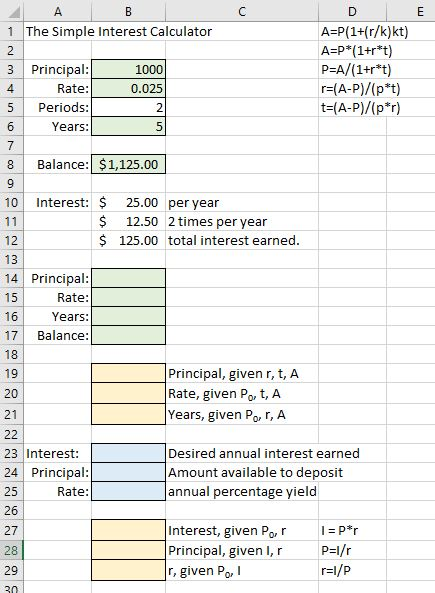2. Into cell B27, type =B24*B25
3. Into cell B28, type =B23/B25
4. Into cell B29, type =B23/B24
5. Now we have the functionality to speculate about the annual simple interest earned given the interest we desire, the principal we have available, and/or the available annual percentage yield. You can test your formulas by using the information we know to be true. Enter 25 into cell B23 and 0.025 into cell B25. This indicates that we wish to earn $25 per year at an interest rate of 2.5%. Cell B28 should let us know that we’ll need to deposit$1000. Then enter 25 into cell B23 and 1000 into cell B24. Cell B29 should let us know that we’ll need to obtain a rate of 0.025 in order to satisfy those needs.

The maturity term for a Treasury bond is 30 years. Suppose you decide to purchase a Treasury bond as part of your retirement planning. Bonds pay an annual yield of 4.0%  and issue interest coupons every six months until they mature, at which time you are paid back the face value of the bond. You’ve been saving money and working hard and have a little cash to invest. You decide to purchase $5,000 in bonds and hold them for 30 years. (a) How much interest are you paid each year? (b) How much is each interest coupon? (c) How much interest will you have earned in total when it matures in 30 years? Let’s say you have a little more than$5000 to invest and want to investigate your options.